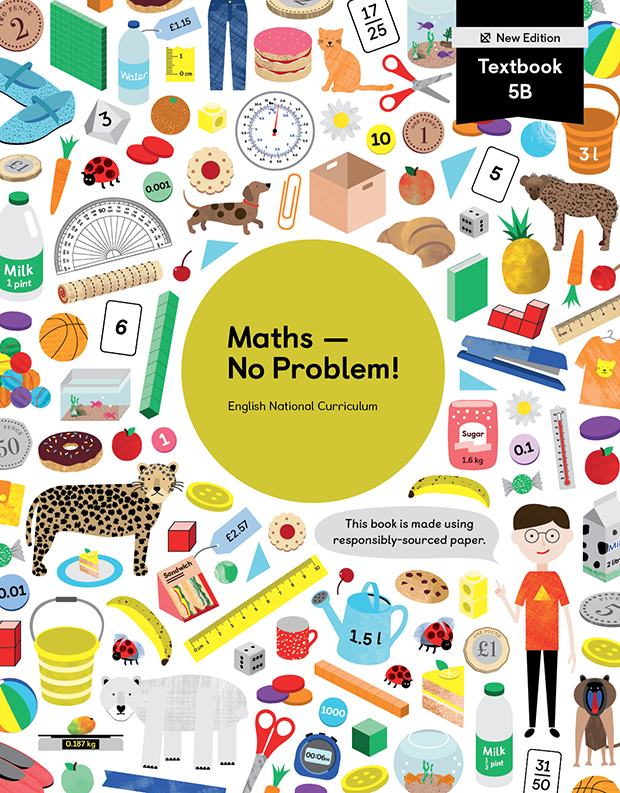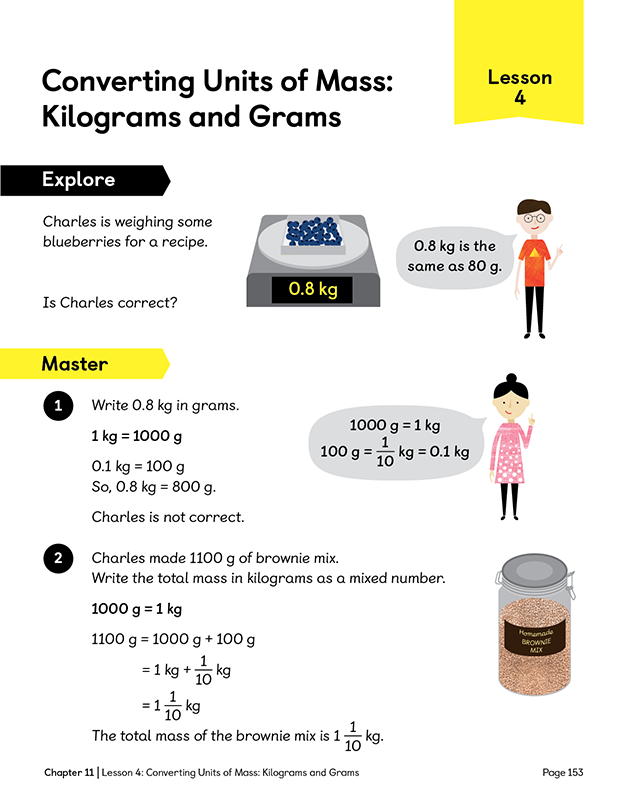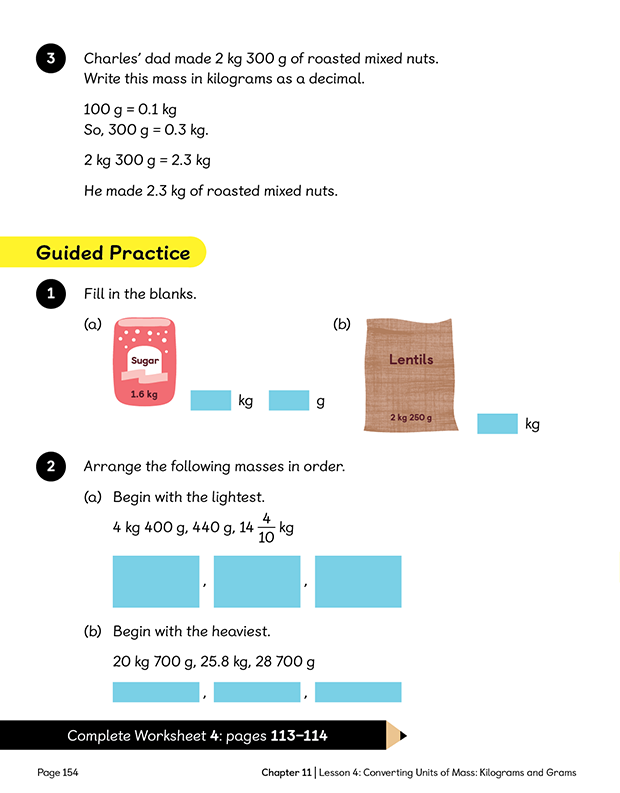Textbook 5B New Edition# Maths — No Problem!Textbook 5B New Edition

## £11.49

ISBN: 9781913458645
Pages: 226
Availability:
In stock
Qty:

• Lesson 1 - Writing DecimalsTo be able to write decimal numbers.
• Lesson 2 - Reading and Writing DecimalsTo be able to read and write decimals.
• Lesson 3 - Reading and Writing DecimalsTo be able to read and write decimals.
• Lesson 4 - Comparing DecimalsTo be able to compare tenths and hundredths written as decimals.
• Lesson 5 - Comparing DecimalsTo be able to order and compare decimals.
• Lesson 6 - Comparing DecimalsTo be able to compare and order decimals.
• Lesson 7 - Writing Fraction and Decimal EquivalentsTo be able to write fractions as decimals.
• Lesson 8 - Adding and Subtracting DecimalsTo be able to add and subtract decimals with one decimal place.
• Lesson 9 - Adding and Subtracting DecimalsTo be able to add and subtract decimals with up to 2 decimal places. To be able to add and subtract amounts in pounds and pence.
• Lesson 10 - Adding and Subtracting DecimalsTo be able to add and subtract amounts in pounds and pence when no renaming is required.
• Lesson 11 - Adding and Subtracting DecimalsTo be able to add and subtract decimals with up to 2 decimal places. To be able to add and subtract amounts in pounds and pence.
• Lesson 12 - Adding and Subtracting DecimalsTo be able to add and subtract decimals with up to 3 decimal places.
• Lesson 13 - Adding and Subtracting DecimalsTo be able to add and subtract decimals. To find number pairs that add up to 1.
• Lesson 14 - Adding and Subtracting DecimalsTo apply knowledge of decimals in real-world problems, such as the perimeter of shapes.
• Lesson 15 - Rounding DecimalsTo be able to round decimals to the nearest whole number. To be able to round numbers to the nearest tenth.
• Chapter ConsolidationTo be able to apply knowledge of decimals to solve problems.

## Author(s)

Consultant Editors: Dr Wong Khoon Yoong, Dr Julie Alderton
Series Editors: Adam Gifford, Andy Psarianos